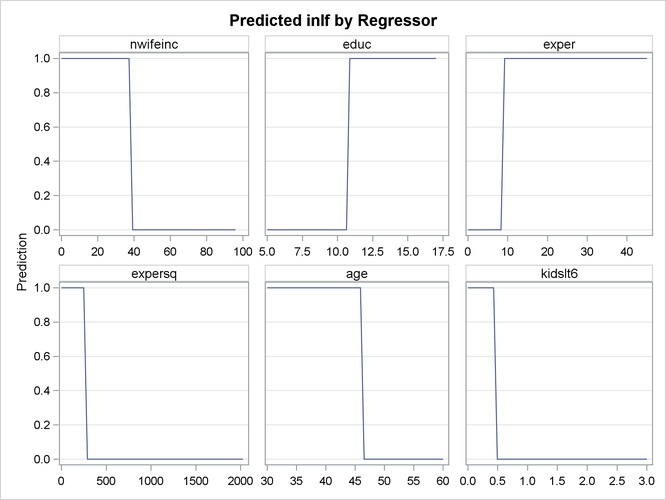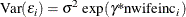# The QLIM Procedure

### Introductory Example: Binary Probit and Logit Models

The following example illustrates the use of PROC QLIM. The data were originally published by Mroz (1987) and downloaded from Wooldridge (2002). This data set is based on a sample of 753 married white women. The dependent variable is a discrete variable of labor force participation (inlf ). Explanatory variables are the number of children ages 5 or younger (kidslt6 ), the number of children ages 6 to 18 (kidsge6 ), the woman’s age (age ), the woman’s years of schooling (educ ), wife’s labor experience (exper ), square of experience (expersq ), and the family income excluding the wife’s wage (nwifeinc ). The program (with data values omitted) is as follows:

/*-- Binary Probit --*/
proc qlim data=mroz plots=predicted;
model inlf = nwifeinc educ exper expersq
age kidslt6 kidsge6  / discrete;
run;


Results of this analysis are shown in the following four figures. In the first table, shown in Figure 22.1, PROC QLIM provides frequency information about each choice. In this example, 428 women participate in the labor force (inlf =1).

Figure 22.1: Choice Frequency Summary

 Binary Data

The QLIM Procedure

Discrete Response Profile of inlf
Index Value Total Frequency
1 0 325
2 1 428

The second table is the estimation summary table shown in Figure 22.2. Included are the number of dependent variables, names of dependent variables, the number of observations, the log-likelihood function value, the maximum absolute gradient, the number of iterations, AIC, and Schwarz criterion.

Figure 22.2: Fit Summary Table of Binary Probit

Model Fit Summary
Number of Endogenous Variables 1
Endogenous Variable inlf
Number of Observations 753
Log Likelihood -401.30219
Maximum Absolute Gradient 0.0000669
Number of Iterations 15
Optimization Method Quasi-Newton
AIC 818.60439
Schwarz Criterion 855.59691

Goodness-of-fit measures are displayed in Figure 22.3. All measures except McKelvey-Zavoina’s definition are based on the log-likelihood function value. The likelihood ratio test statistic has chi-square distribution conditional on the null hypothesis that all slope coefficients are zero. In this example, the likelihood ratio statistic is used to test the hypothesis that kidslt6 =kidge6 =age =educ =exper =expersqnwifeinc = 0.

Figure 22.3: Goodness of Fit

Goodness-of-Fit Measures
Measure Value Formula
Likelihood Ratio (R) 227.14 2 * (LogL - LogL0)
Upper Bound of R (U) 1029.7 - 2 * LogL0
Aldrich-Nelson 0.2317 R / (R+N)
Cragg-Uhler 1 0.2604 1 - exp(-R/N)
Cragg-Uhler 2 0.3494 (1-exp(-R/N)) / (1-exp(-U/N))
Estrella 0.2888 1 - (1-R/U)^(U/N)
Adjusted Estrella 0.2693 1 - ((LogL-K)/LogL0)^(-2/N*LogL0)
McFadden's LRI 0.2206 R / U
Veall-Zimmermann 0.4012 (R * (U+N)) / (U * (R+N))
McKelvey-Zavoina 0.4025

The parameter estimates and standard errors are shown in Figure 22.4.

Figure 22.4: Parameter Estimates of Binary Probit

Parameter Estimates
Parameter DF Estimate Standard
Error
t Value Approx
Pr > |t|
Intercept 1 0.270077 0.508590 0.53 0.5954
nwifeinc 1 -0.012024 0.004840 -2.48 0.0130
educ 1 0.130905 0.025255 5.18 <.0001
exper 1 0.123348 0.018720 6.59 <.0001
expersq 1 -0.001887 0.000600 -3.14 0.0017
age 1 -0.052853 0.008477 -6.24 <.0001
kidslt6 1 -0.868329 0.118519 -7.33 <.0001
kidsge6 1 0.036005 0.043477 0.83 0.4076

Finally, the QLIM procedure profiles the predicted outcome with respect to the regressors. For example, Figure 22.5 shows the predicted values profiled with respect to nwifeinc, educ, exper, expersq, age, and kidslt6.

Figure 22.5: Predictions by Regressors: nwifeinc, educ, exper, expersq, age, and kidslt6When the error term has a logistic distribution, the binary logit model is estimated. To specify a logistic distribution, add D=LOGIT option as follows:

/*-- Binary Logit --*/
proc qlim data=mroz;
model inlf = nwifeinc educ exper expersq
age kidslt6 kidsge6  / discrete(d=logit);
run;


The estimated parameters are shown in Figure 22.6.

Figure 22.6: Parameter Estimates of Binary Logit

 Binary Data

The QLIM Procedure

Parameter Estimates
Parameter DF Estimate Standard
Error
t Value Approx
Pr > |t|
Intercept 1 0.425452 0.860365 0.49 0.6210
nwifeinc 1 -0.021345 0.008421 -2.53 0.0113
educ 1 0.221170 0.043441 5.09 <.0001
exper 1 0.205870 0.032070 6.42 <.0001
expersq 1 -0.003154 0.001017 -3.10 0.0019
age 1 -0.088024 0.014572 -6.04 <.0001
kidslt6 1 -1.443354 0.203575 -7.09 <.0001
kidsge6 1 0.060112 0.074791 0.80 0.4215

The heteroscedastic logit model can be estimated using the HETERO statement. If the variance of the logit model is a function of the family income level excluding wife’s income (nwifeinc ), the variance can be specified aswhereis normalized to 1 because the dependent variable is discrete. The following SAS statements estimate the heteroscedastic logit model:

/*-- Binary Logit with Heteroscedasticity --*/
proc qlim data=mroz;
model inlf = nwifeinc educ exper expersq
age kidslt6 kidsge6 / discrete(d=logit);
hetero inlf ~ nwifeinc / noconst;
run;


The parameter estimate,, of the heteroscedasticity variable is listed as _H.nwifeinc; see Figure 22.7.

Figure 22.7: Parameter Estimates of Binary Logit with Heteroscedasticity

 Binary Data

The QLIM Procedure

Parameter Estimates
Parameter DF Estimate Standard
Error
t Value Approx
Pr > |t|
Intercept 1 0.510445 0.983538 0.52 0.6038
nwifeinc 1 -0.026778 0.012108 -2.21 0.0270
educ 1 0.255547 0.061728 4.14 <.0001
exper 1 0.234105 0.046639 5.02 <.0001
expersq 1 -0.003613 0.001236 -2.92 0.0035
age 1 -0.100878 0.021491 -4.69 <.0001
kidslt6 1 -1.645206 0.311296 -5.29 <.0001
kidsge6 1 0.066941 0.085633 0.78 0.4344
_H.nwifeinc 1 0.013280 0.013606 0.98 0.3291